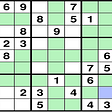# Prefix sum + two layer loops.

• Calculate prefix sum for each column
• for all possible combinations of row ranges, check the maximum possible submatrix after rearrangement.
`class Solution {public:    int largestSubmatrix(vector<vector<int>>& a) {        const int R = a.size();        const int C = a.size();       // cout<<R<<" "<<C<<endl;        int ret = 0;        int this_ret = 0;        // quick check when num of column is 1 to get rid of TLE        if (C == 1) {            for (int i =0; i < R;++i){                if (a[i]){                    this_ret++;                } else {                    ret = max(ret, this_ret);                    this_ret = 0;                }            }             ret = max(ret, this_ret);            return ret;        }                       for (int j = 0; j < C; ++j) {            for (int i = 1; i < R; ++i){                a[i][j] += a[i-1][j];            }        }              int count = 0;        for (int i = 0; i < R; ++i) {            for (int j = i; j < R; ++j) {                count = 0;                int n = j- i +1;                // early pruning to get rid of TLE                if (n*C <= ret) continue;                for (int k = 0; k < C; ++k) {                    if (i==0 && a[j][k]==n) count++;                    if (i!=0 && a[j][k] - a[i-1][k]==n)count++;                }                ret = max(ret, count*n);            }        }        return ret;    }};`

# A better code from int65536

`class Solution{public:    int largestSubmatrix(vector<vector<int>>& a)  {  int n = a.size();  int m = a.size();  vector<int> count(m);  int result = 0;  for (int i = 0; i < n; ++i) {   for (int j = 0; j < m; ++j) {    count[j] = (a[i][j] ? count[j] + 1 : 0);   }   vector<int> b = count;   sort(b.begin(), b.end());   reverse(b.begin(), b.end());   for (int j = 0; j < m; ++j) {    result = max(result, (j + 1) * b[j]);   }  }  return result; }};`
`//1) get the number of continuous 1s up to the row icount[j] = (a[i][j] ? count[j] + 1 : 0);//2) sort the count reversely -> from largest to smallest sort(b.begin(), b.end());reverse(b.begin(), b.end());//3) loop the column and harvest the results. As j is from 0, we should add j by 1.(j + 1) * b[j]`

--

--

--

## More from Jimmy Shen

Data Scientist/MLE/SWE @takemobi

Love podcasts or audiobooks? Learn on the go with our new app.

## ADD.XYZ’s Weekly Update 30 — Google Apps, Strategic Deals and more..## CS373 Spring 2021: Software Engineering — Week 4## SQL DELETE Statement## Read Routing Features for Always-On Server## How To Compress Images Using AWS Lambda## Ixian Progress Report## Jimmy Shen

Data Scientist/MLE/SWE @takemobi

## Scaling and gradient descent optimization in neural network## BACKTRACKING ALGORITHM## Control of the Generalization Error in Statistical Learning theory (part 2)